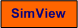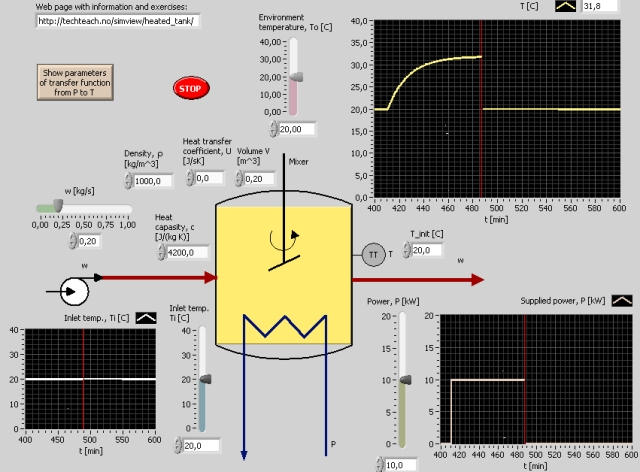# Heated Tank

Snapshot of the front panel of the simulator:• What is needed to run the the simulator? Read to get most recent information!
• Tips for using the simulator.
• The simulator: . The simulator runs immediately after the download by clicking Open in the download window. Alternatively, you can first save a copy of the exe-file on any directory (folder) on your PC and then run the exe-file, which starts the simulator.

## Description of the simulated system

In this simulator a thermal system is simulated. The system is a continuously stirred heated tank with continuous mass (liquid) inflow and outflow. The front panel of the simulator shows the system. The mathematical model can be derived from an energy balance for the liquid in the tank. The energy balance can be expressed as the following first order differential equation:

crVdT/dt = P + cw(Ti - T) + U(To - T)

der

• T [oC] is the liquid temperature in the tank
• Ti [oC]  is the inlet temperature
• To [oC]  is the ambient temperature
• c [J/(kg K)] is the specific heat capacity of the liquid
• r [kg/m3] is the liquid density.
• w [kg/s] is the liquid mass flow through the tank
• V [m3] is the tank volume
• U [J/(s K)] is the total heat transfer coefficient (between the the tank and the environment)

The simulator is based on the model above.

## Aims

The aim of the simulator and the tasks below is to develop the understanding of the dynamic properties of a continuously stirred heated tank with continuous heating and inlet flow and outlet flow. This system .

## Motivation

Stirred tanks are common in industrial plants. The dynamic properties of heated tanks, heat exchangers, and reactors have many similarities with stirred tanks.

Unless otherwise stated, you should use the default values of the parameters (a default value is retrieved via right-click on the element / Reinitialize to Default on the front panel).

1. Get familiar with the system/model: Observe in simulations how the temperature T of the liquid in the tank depends qualitatively of the following variables and parameters:
• Inlet temperature Ti (it can be changed e.g. as a step)
• Mass flow w (e.g. increase it).
• Environmental temperature Ti.
• Heat transfer coefficient U (increase from e.g. U=500).

2. Static operating point: It is specified that the static value of the tank temperature, Ts, shall be 40 degrees C. The inlet flow temperature is assumed to be Ti0  = 20 degrees C. Calculate from the static version of the model the constant power, P0, neeeded. In this case use the default values of the prosess parameters (as shown on the frontpanel). Confirm the calculated resultat in a simulation.

[SimView] [TechTeach]

Updated 10 September 2017. Developed by Finn Haugen. E-mail: finn@techteach.no.